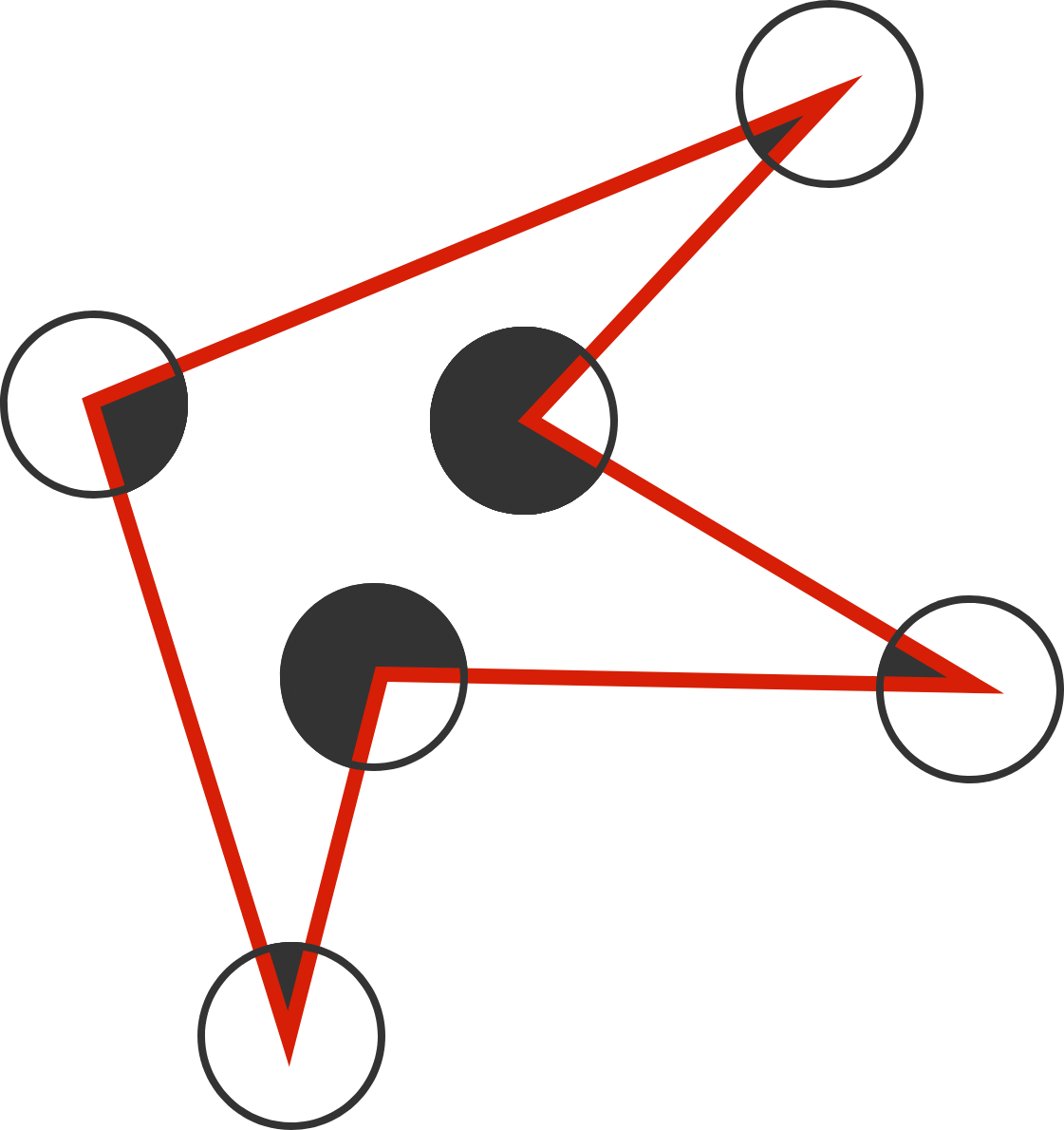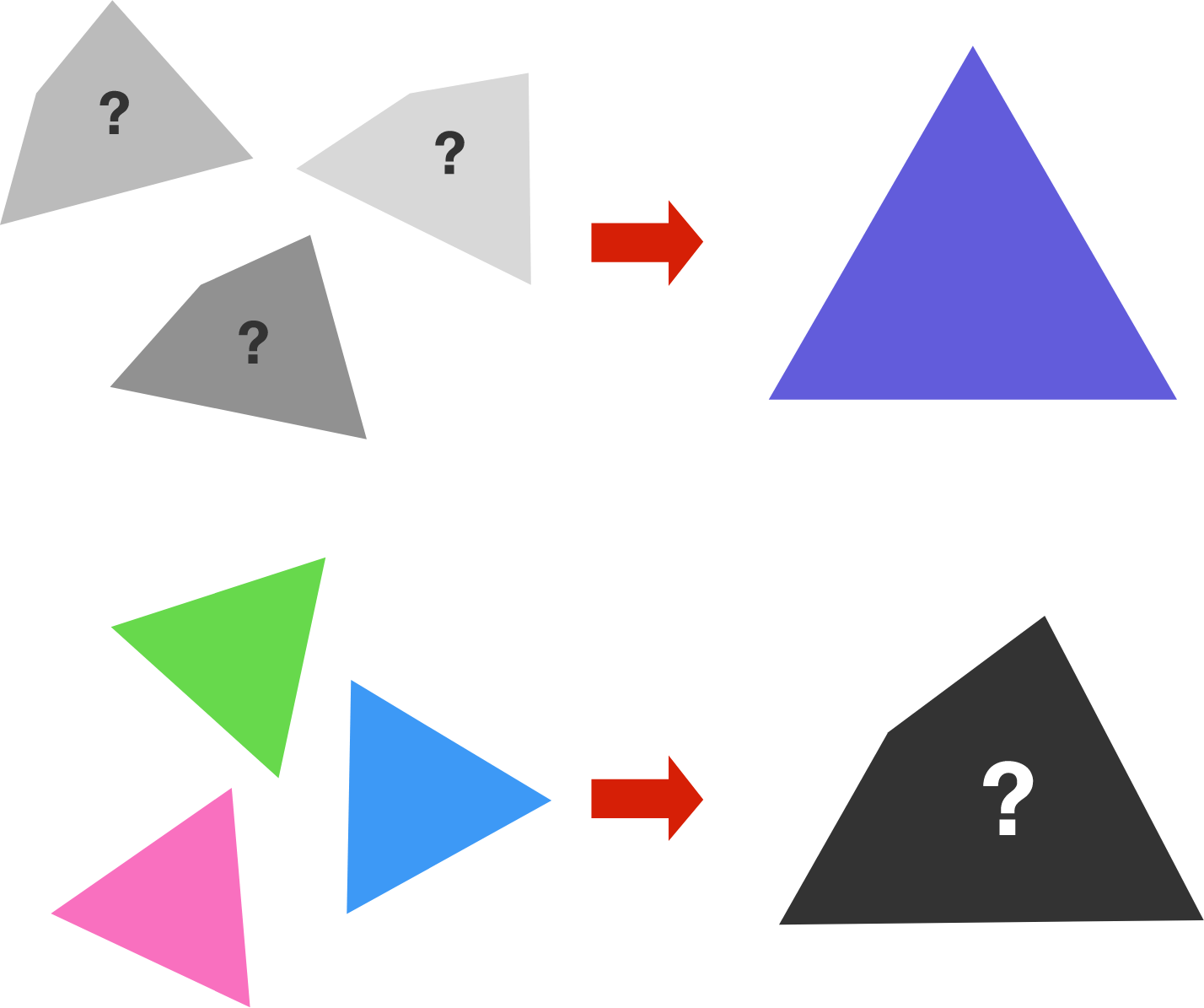Geometry

General Polygons: Level 2 ChallengesThe figure to the right shows an irregular hexagon with six circles of radius 1, where the hexagon's vertices are the circles' centers.

Find the sum of the areas of the black regions.

True or False?

$\quad$ Statement I: If all sides of a polygon are equal, then all angles of the polygon are equal.

$\quad$ Statement II: If all angles of a polygon are equal, then all sides of the polygon are equal.I am a peculiar quadrilateral. If you put 3 copies of me together, then I can form an equilateral triangle. Similarly, if you put 3 copies of an equilateral triangle together, then they can form me!